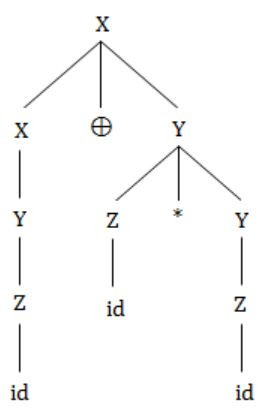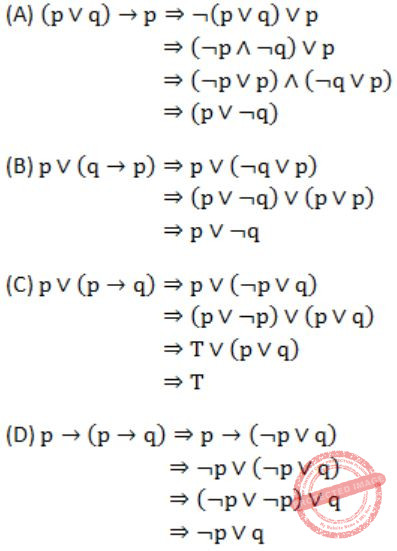## GATE 1997

 Question 1

The probability that it will rain today is 0.5. The probability that it will rain tomorrow is 0.6. The probability that it will rain either today or tomorrow is 0.7. What is the probability that it will rain today and tomorrow?

 A 0.3 B 0.25 C 0.35 D 0.4
Engineering-Mathematics       Probability
Question 1 Explanation:
P(Today) = 0.5
P(Tomorrow) = 0.6
P(T∪To) = 0.7
P(T∩To) = P(T) + P(To) - P(T∪To)
= 0.5 + 0.6 - 0.07
= 1.1 - 0.7
= 0.4
 Question 2

The Newton-Raphson method is used to find the root of the equation x2 - 2 = 0. If the iterations are started from -1, the iterations will

 A converge to -1 B converge to √2 C converge to - √2 D not converge
Engineering-Mathematics       Newton-Raphson-Method
Question 2 Explanation:Question 3

The determinant of the matrix isis:

 A 11 B -48 C 0 D -24
Engineering-Mathematics       Linear-Algebra
Question 3 Explanation:
Determinant of given matrix = 6 × 2 × 4 × (-1) = -48
 Question 4

The concatenation of two lists is to be performed in O(1) time. Which of the following implementations of a list should be used?

 A Singly linked list B Doubly linked list C Circular doubly linked list D Array implementation of list
Question 4 Explanation:
In circular doubly linked list concatenation of two lists is to be performed on O(1) time.
 Question 5

The correct matching for the following pairs is

```(A) All pairs shortest path          (1) Greedy
(B) Quick Sort                       (2) Depth-First search
(C) Minimum weight spanning tree     (3) Dynamic Programming
(D) Connected Components             (4) Divide and and Conquer ```
 A A – 2 B – 4 C – 1 D – 3 B A – 3 B – 4 C – 1 D – 2 C A – 3 B – 4 C – 2 D – 1 D A – 4 B – 1 C – 2 D – 3
Algorithms        Match-the-Following
Question 5 Explanation:
All pairs shortest path - Dynamic Programming
Quick sort - Divide and Conquer
Minimum weight Spanning tree - Greedy
Connected components - Depth-First search
 Question 6

In the following grammar

```         X ::= X ⊕ Y/Y
Y ::= Z * Y/Z
Z ::= id  ```

Which of the following is true?

 A ‘⊕’ is left associative while ‘*’ is right associative B Both ‘⊕’ and ‘*’ is left associative C ‘⊕’ is right associative while ‘*’ is left associative D None of the above
Compiler-Design       Grammar
Question 6 Explanation:⊕ is left associative.
* is right associative.
 Question 7

Which of the following is essential for converting an infix expression to the postfix from efficiently?

 A An operator stack B An operand stack C An operand stack and an operator stack D A parse tree
Data-Structures       Stacks
Question 7 Explanation:
An operator stack ⇒ Infix to (Postfix or Prefix)
An operand stack ⇒ Postfix to Prefix
Operator & operand stack ⇒ We don't use two stacks
Parse tree ⇒ No use
 Question 8

A language L allows declaration of arrays whose sizes are not known during compilation. It is required to make efficient use of memory. Which of the following is true?

 A A compiler using static memory allocation can be written for L B A compiler cannot be written for L; an interpreter must be used C A compiler using dynamic memory allocation can be written for L D None of the above
Compiler-Design       Run-Time-Environments
Question 8 Explanation:
Compiler is use dynamic memory allocation then the memory will be allocated to an array at runtime.
 Question 9

The conditional expansion facility of macro processor is provided to

 A test a condition during the execution of the expanded program B to expand certain model statements depending upon the value of a condition during the execution of the expanded program C to implement recursion D to expand certain model statements depending upon the value of a condition during the process of macro expansion
Compiler-Design       Macros
Question 9 Explanation:
Macro is expanded during the process of Macro expansion.
 Question 10

Heap allocation is required for languages

 A that support recursion B that support dynamic data structures C that use dynamic scope rules D None of the above
Compiler-Design       Run-Time-Environments
Question 10 Explanation:
Heap allocation is required for languages that support dynamic data structures.
 Question 11

Let * be defined as x * y = x' + y. Let z = x * y. Value of z * x is

 A x'+y B x C 0 D 1
Digital-Logic-Design       Boolean-Expressions
Question 11 Explanation:Question 12

RST 7.5 interrupt in 8085 microprocessor executes the interrupt service routine from interrupt vector location

 A 0000H B 0075H C 003CH D 0034H
Computer-Organization       Microprocessor
Question 12 Explanation:
RST7.5 then location is = 7.5*8 = 60 (8085 is 8 bit processor)
→ 60 in hexa decimal is 003CH.
 Question 13

Purpose of a start bit in RS 232 serial communication protocol is

 A to synchronize receiver for receiving every byte B to synchronize receiver for receiving a sequence of bytes C a parity bit D to synchronize receiver for receiving the last byte
Computer-Organization       Serial-Communication
Question 13 Explanation:
RS-232 needs a start before each byte transmission for synchronization.
 Question 14

The correct matching for the following pairs is

```(A) DMA I/O                    (1) High speed RAM
(B) Cache                      (2) Disk
(C) Interrupt I/O              (3) Printer
(D) Condition Code Register    (4) ALU ```
 A A – 4 B – 3 C – 1 D – 2 B A – 2 B – 1 C – 3 D – 4 C A – 4 B – 3 C – 2 D – 1 D A – 2 B – 3 C – 4 D – 1
Computer-Organization       Match-the-Following
Question 14 Explanation:
DMA I/O → Disk
Cache → High speed RAM
Interrupt I/O → Printer
Condition code register → ALU
 Question 15

An N-bit carry look ahead adder, where N is a multiple of 4, employs ICs 74181 (4 bit ALU) and 74182 (4 bit carry look ahead generator).

The minimum addition time using the best architecture for this adder is

 A proportional to N B proportional to log N C a constant D None of the above
 Question 16

Let (Z,*) be an algebraic structure, where Z is the set of integers and the operation * is defined by n*m = maximum (n,m). which of the following statements is true for (Z,*)?

 A (Z,*) is a monoid B (Z,*) is an Abelian group C (Z,*) is a group D None of the above
Engineering-Mathematics       Sets-And-Relation
Question 16 Explanation:
Semigroup - Closed and associative
Monoid - Closed, Associative and has an identity
Group - Monoid with inverse
Abelian group - Group with commutative property
Go through with given:
Closure: Yes.
(m*n = max(m,n)) output is either m or n whichever is maximum since m,n belongs z. The result of the binary operation also belongs to z. So given is satisfying closure property.
Associative: Yes.
The output is max among the elements and it is associative.
Identity: No.
We don't have single unique element for all the elements which is less than all the elements.
Given one is semigroup only.
 Question 17

Which of the following propositions is a tautology?

 A (p ∨ q) → p B p ∨ (q → p) C p ∨ (p → q) D p → (p → q)
Engineering-Mathematics       Propositional-Logic
Question 17 Explanation:Question 18

In a lattice defined by the Hasse diagram given in following figure, how many complements does the element 'e' have?A 2 B 3 C 0 D 1
Engineering-Mathematics       Sets-And-Relation
Question 18 Explanation:
g, c, d are the complements of e.
No. of complements = 3
e∨g = a and e∧g = f
e∨c = a and e∧c = f
e∨d = a and e∧d = f
There are 18 questions to complete.

Register Now